# Bohr’s atomic model

Neils Bohr proposed a model of an atom based on atomic spectra of hydrogen.

He retained the basic concepts of Rutherford’s model i.e., the atom has a positively charged nucleus at the center and the electrons revolve around the nucleus. He applied Planck’s Quantum theory for revolving electron.

The main postulates are:

Electrons revolve around the nucleus with high velocity in circular paths called orbits or shells.

As long as the electron is in a particular orbit, its energy is constant. Therefore, these orbits are called stationary orbits.

Each stationary orbit is associated with a definite energy and is known as energy level. These energy levels are named as K, L, M, N, ….. etc.  or numbered as 1,2,3,4… etc.

When an electron jumps from a lower energy level to a higher energy level, the difference in energy is emitted as radiation in quanta.

When an electron jumps from a lower energy level to a higher energy level, the difference in energy is absorbed as radiation in quanta.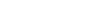where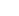energy of first orbit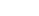energy of second orbit

h = Planck’s constant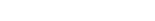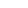frequency of radiation

The angular momentum of the electron revolving in a stationary orbit is equal to integral multiples of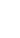.

Angular momentum,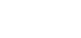where

n = integer (1,2,3,4…)

h = Planck’s constant

m = mass of the electron

v = velocity of electron

r = radius of the circular orbit

Therefore, the angular momentum of the electron in various orbits is equal to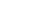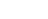etc.

Thus, the momentum is said to be quantized.

Merits of Bohr’s atomic model:

• This theory explains the various spectral series of hydrogen atom in excellent manner.
• This theory also explains the spectral series of ions like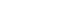etc.
• This theory is highly successful in the aspect of calculating the radius and the energy of each orbit of hydrogen atom.

Limitations of Bohr’s atomic model :

• Bohr’s atomic theory could not explain the spectra of atoms having more than one electron. Examples: He, Li, Be, B, C.
• This theory doesn’t explain splitting of spectral lines into a group of lines under the influence of magnetic field and electric field.
• This theory could not justify the Quantization of angular momentum.
• This theory could not account for the formation of chemical bonds.
• This theory assumes electron as a particle. This is against the wave nature of electron proposed by de Broglie.
• This theory gives the position and velocity of electron accurately and simultaneously. This is against the uncertainty principle proposed by Heisenberg.